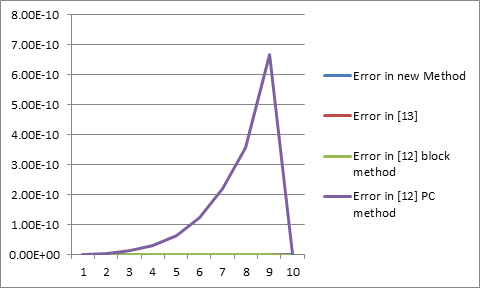# Numerical Application of Higher Order Linear Block Method for Solving Some Fourth Order Initial Value Problems

## Authors

• D. Raymond Department of Mathematics and Statistic, Federal University Wukari, Nigeria
• T. Y. Kyagya Department of Mathematics and Statistic, Federal University Wukari, Nigeria
• S. John Department of Mathematics, Adamawa University Mubi, Nigeria
• A. Lydia Department of Mathematics and Statistic, Federal University Wukari, Nigeria

## Keywords:

Numerical application, Block hybrid method, Initial value problems, Differential equation

## Abstract

The numerical application of higher order linear block method for the direct solution of fourth order initial value problems was proposed using the linear block algorithm, where the methods applied in block form. The method is zero-stabile, consistent and convergent when analyzing the properties of the method. The mathematical example solved using the method is effective, suitable, and acceptable for solving fourth order initial value problems. The method is also compared with existing work when solving similar systems of differential equation and obviously, the method performs better than those in literature and textual shown.

Dimensions

J. Sabo, “Single step block hybrid methods for the direct solution of higher order initial value problems”, M.Sc. Adamawa State University, Mubi (Unpublished) (2021) 9.

J. Kuboye, O. R. Elusakin & O. F. Quadri, “Numerical algorithm for direct solution of fourth order ordinary differential equations”, Journal of Nigerian Society of Physical Sciences 2 (2020) 218. https//doi.org/10.46481/jnsps.2020.100

O. Adeyeye & Z. Omar, “A new algorithm for developing block methods for solving fourth order ordinary differential equations”, Global Journal of Pure and Applied Mathematics 12 (2016) 1466.

J. O. Kuboye & Z. Omar, “New Zero-stable block method for direct solution of fourth order ordinary differential equations”, Indian Journal of Science and Technology 8 (2015) 1.

J. Sabo, Y. Skwame & J. Z. Donald, “On the simulation of higher order linear block algorithm for modelling fourth order initial value problems”, Asian Research Journal of Mathematics 18 (2022) 23.

E. A. Areo & E. O. Omole, “Half-step symmetric continous hybrid block method for the numerical solutions of fourth order ordinary differential equations”, Arch. Appl. Sci. Res. 7 (2015) 40.

J. D. Lambert, Computational Methods in ODEs, John Wiley & Sons, New York (1973).

S. O. Fatunla, “Numerical Methods for Initial Value Problems in Ordinary Differential Equations”, Academic Press Inc. Harcourt Brace, New York (1991).

D. O. Awoyemi, “Algorithmic collocation methods for the direct solution of special and general fourth order initial value problems in ordinary differential equations”, Inter. Journal of Computer Math. 6 (2001) 271.

J. Kayode, M. Duromola & B. Bolaji, “Direct solution of initial value problems of fourth order ordinary differential equations using modified implicit hybrid block method”, Journal of Scientific Research and Reports 3 (2014) 2793. https//10.9734/jsrr/2014/11953

V. O. Atabo, “A new special 15-step block method for general fourth order ordinary differential equations”, Journal of the Nigerian society of Physical Sciences 3 (2021) 308. https//10.46481/jnsps.2021.337

A. Adeyeye & Z. Omar, “Implicit five-step block method with generalised equidistant points for solving fourth order linear and non-linear initial value problems”, Ain Shams Engineering Journal 10 (2019) 885.

A. D. Familua & E. O. Omole, “Five points mono hybrid point linear multistep method for solving nth order ordinary differential equations using power series function”, Asian Research Journal of Mathematics 3 (2017) 13.

E. A. Areo & E. O. Omole, “Half-Step symmetric continous hybrid block method for the numerical solutions of fourth order ordinary differential equations”, Archives of Applied Science Research 7 (2015) 40.2023-02-04

## How to Cite

Raymond, D., Kyagya, T. Y., John, S., & Lydia, A. (2023). Numerical Application of Higher Order Linear Block Method for Solving Some Fourth Order Initial Value Problems. African Scientific Reports, 2(1), 67. https://doi.org/10.46481/asr.2023.2.1.67

## Section

Original Research